Related Articles

# How to loop through each row of dataFrame in PySpark ?

• Last Updated : 25 Aug, 2021

In this article, we are going to see how to loop through each row of Dataframe in PySpark. Looping through each row helps us to perform complex operations on the RDD or Dataframe.

Creating Dataframe for demonstration:

## Python3

 `# importing necessary libraries``import` `pyspark``from` `pyspark.sql ``import` `SparkSession` `# function to create new SparkSession``def` `create_session():``    ``spk ``=` `SparkSession.builder \``        ``.master(``"local"``) \``        ``.appName(``"employee_profile.com"``) \``        ``.getOrCreate()``    ``return` `spk`  `def` `create_df(spark, data, schema):``    ``df1 ``=` `spark.createDataFrame(data, schema)``    ``return` `df1`  `if` `__name__ ``=``=` `"__main__"``:` `    ``# calling function to create SparkSession``    ``spark ``=` `create_session()` `    ``input_data ``=` `[(``1``, ``"Shivansh"``, ``"Data Scientist"``, ``"Noida"``),``                  ``(``2``, ``"Rishabh"``, ``"Software Developer"``, ``"Banglore"``),``                  ``(``3``, ``"Swati"``, ``"Data Analyst"``, ``"Hyderabad"``),``                  ``(``4``, ``"Amar"``, ``"Data Analyst"``, ``"Noida"``),``                  ``(``5``, ``"Arpit"``, ``"Android Developer"``, ``"Pune"``),``                  ``(``6``, ``"Ranjeet"``, ``"Python Developer"``, ``"Gurugram"``),``                  ``(``7``, ``"Priyanka"``, ``"Full Stack Developer"``, ``"Banglore"``)]` `    ``schema ``=` `[``"Id"``, ``"Name"``, ``"Job Profile"``, ``"City"``]` `    ``# calling function to create dataframe``    ``df ``=` `create_df(spark, input_data, schema)` `    ``# retrieving all the elements of``    ``# the dataframe using collect()``    ``# Storing in the variable``    ``data_collect ``=` `df.collect()` `    ``df.show()`

Output: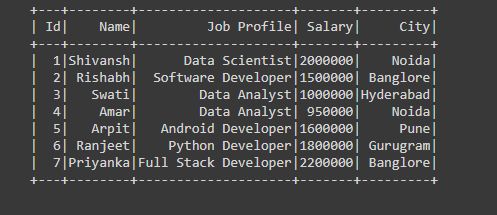### Method 1: Using collect()

We can use collect() action operation for retrieving all the elements of the Dataset to the driver function then loop through it using for loop.

## Python3

 `# retrieving all the elements``# of the dataframe using collect()``# Storing in the variable``data_collect ``=` `df.collect()` `# looping thorough each row of the dataframe``for` `row ``in` `data_collect:``    ``# while looping through each``    ``# row printing the data of Id, Name and City``    ``print``(row[``"Id"``],row[``"Name"``],``"  "``,row[``"City"``])`

Output: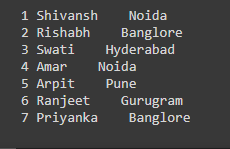### Method 2: Using toLocalIterator()

We can use toLocalIterator(). This returns an iterator that contains all the rows in the DataFrame. It is similar to collect(). The only difference is that collect() returns the list whereas toLocalIterator() returns an iterator.

## Python

 `data_itr ``=` `df.rdd.toLocalIterator()` `# looping thorough each row of the dataframe``for` `row ``in` `data_itr:``  ` `    ``# while looping through each row printing``    ``# the data of Id, Job Profile and City``    ``print``(row[``"Id"``],``" "``,row[``"Job Profile"``],``"  "``,row[``"City"``])`

Output: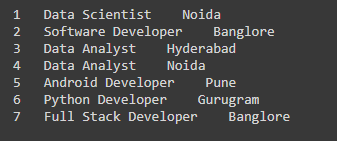Note: This function is similar to collect() function as used in the above example the only difference is that this function returns the iterator whereas the collect() function returns the list.

### Method 3: Using iterrows()

The iterrows() function for iterating through each row of the Dataframe, is the function of pandas library, so first, we have to convert the PySpark Dataframe into Pandas Dataframe using toPandas() function. Then loop through it using for loop.

## Python

 `pd_df ``=` `df.toPandas()` `# looping through each row using iterrows()``# used to iterate over dataframe rows as index,``# series pair``for` `index, row ``in` `pd_df.iterrows():``  ` `    ``# while looping through each row``    ``# printing the Id, Name and Salary``    ``# by passing index instead of Name``    ``# of the column``    ``print``(row[``0``],row[``1``],``" "``,row[``3``])`

Output: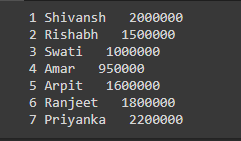### Method 4: Using map()

map() function with lambda function for iterating through each row of Datafarame. For looping through each row using map() first we have to convert the PySpark dataframe into RDD because map() is performed on RDD’s only, so first convert into RDD it then use map() in which, lambda function for iterating through each row and stores the new RDD in some variable then convert back that new RDD into Datafarame using toDF() by passing schema into it.

## Python

 `# importing necessary libraries``import` `pyspark``from` `pyspark.sql ``import` `SparkSession` `# function to create new SparkSession``def` `create_session():``  ``spk ``=` `SparkSession.builder \``      ``.master(``"local"``) \``      ``.appName(``"employee_profile.com"``) \``      ``.getOrCreate()``  ``return` `spk` `def` `create_df(spark,data,schema):``  ``df1 ``=` `spark.createDataFrame(data,schema)``  ``return` `df1` `if` `__name__ ``=``=` `"__main__"``:` `  ``# calling function to create SparkSession``  ``spark ``=` `create_session()``    ` `  ``input_data ``=` `[(``1``,``"Shivansh"``,``"Data Scientist"``,``2000000``,``"Noida"``),``          ``(``2``,``"Rishabh"``,``"Software Developer"``,``1500000``,``"Banglore"``),``          ``(``3``,``"Swati"``,``"Data Analyst"``,``1000000``,``"Hyderabad"``),``          ``(``4``,``"Amar"``,``"Data Analyst"``,``950000``,``"Noida"``),``          ``(``5``,``"Arpit"``,``"Android Developer"``,``1600000``,``"Pune"``),``          ``(``6``,``"Ranjeet"``,``"Python Developer"``,``1800000``,``"Gurugram"``),``          ``(``7``,``"Priyanka"``,``"Full Stack Developer"``,``2200000``,``"Banglore"``)]` `  ``schema ``=` `[``"Id"``,``"Name"``,``"Job Profile"``,``"Salary"``,``"City"``]` `  ``# calling function to create dataframe``  ``df ``=` `create_df(spark,input_data,schema)` `  ``# map() is only be performed on rdd``  ``# so converting the dataframe into rdd using df.rdd``  ``rdd ``=` `df.rdd.``map``(``lambda` `loop: (``      ``loop[``"Id"``],loop[``"Name"``],loop[``"Salary"``],loop[``"City"``])``  ``)` `  ``# after looping the getting the data from each row``  ``# converting back from RDD to Dataframe``  ``df2 ``=` `rdd.toDF([``"Id"``,``"Name"``,``"Salary"``,``"City"``])` `  ``# showing the new Dataframe``  ``df2.show()`

Output: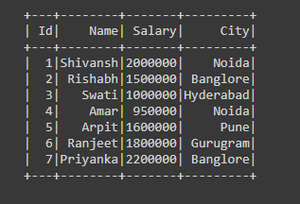### Method 5: Using list comprehension

We can use list comprehension for looping through each row which we will discuss in the example.

## Python

 `# using list comprehension for looping``# through each row  storing the list of``# data in the variable ``table ``=` `[x[``"Job Profile"``] ``for` `x ``in` `df.rdd.collect()]` `# looping the list for printing ``for` `row ``in` `table:``    ``print``(row)`

Output: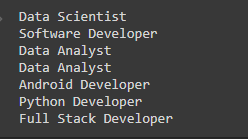### Method 6: Using select()

The select() function is used to select the number of columns. After selecting the columns, we are using the collect() function that returns the list of rows that contains only the data of selected columns.

## Python

 `# importing necessary libraries``import` `pyspark``from` `pyspark.sql ``import` `SparkSession` `# function to create new SparkSession``def` `create_session():``    ``spk ``=` `SparkSession.builder \``        ``.master(``"local"``) \``        ``.appName(``"employee_profile.com"``) \``        ``.getOrCreate()``    ``return` `spk`  `def` `create_df(spark, data, schema):``    ``df1 ``=` `spark.createDataFrame(data, schema)``    ``return` `df1`  `if` `__name__ ``=``=` `"__main__"``:` `    ``# calling function to create SparkSession``    ``spark ``=` `create_session()` `    ``input_data ``=` `[(``1``, ``"Shivansh"``, ``"Data Scientist"``, ``2000000``, ``"Noida"``),``                  ``(``2``, ``"Rishabh"``, ``"Software Developer"``, ``1500000``, ``"Banglore"``),``                  ``(``3``, ``"Swati"``, ``"Data Analyst"``, ``1000000``, ``"Hyderabad"``),``                  ``(``4``, ``"Amar"``, ``"Data Analyst"``, ``950000``, ``"Noida"``),``                  ``(``5``, ``"Arpit"``, ``"Android Developer"``, ``1600000``, ``"Pune"``),``                  ``(``6``, ``"Ranjeet"``, ``"Python Developer"``, ``1800000``, ``"Gurugram"``),``                  ``(``7``, ``"Priyanka"``, ``"Full Stack Developer"``, ``2200000``, ``"Banglore"``)]` `    ``schema ``=` `[``"Id"``, ``"Name"``, ``"Job Profile"``, ``"Salary"``, ``"City"``]` `    ``# calling function to create dataframe``    ``df ``=` `create_df(spark, input_data, schema)` `    ``# getting each row of dataframe containing``    ``# only selected columns Selected columns are``    ``# 'Name' and 'Salary' getting the list of rows``    ``# with selected column data using collect()``    ``rows_looped ``=` `df.select(``"Name"``, ``"Salary"``).collect()` `    ``# printing the data of each row``    ``for` `rows ``in` `rows_looped:``      ` `        ``# here index 0 and 1 refers to the data``        ``# of 'Name' column and 'Salary' column``        ``print``(rows[``0``], rows[``1``])`

Output: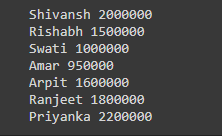Attention geek! Strengthen your foundations with the Python Programming Foundation Course and learn the basics.

To begin with, your interview preparations Enhance your Data Structures concepts with the Python DS Course. And to begin with your Machine Learning Journey, join the Machine Learning – Basic Level Course

My Personal Notes arrow_drop_up